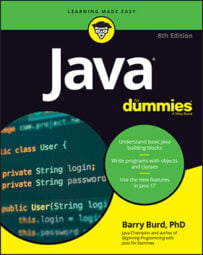##### Java For DummiesYou can use method references in Java. Here’s an example. Taken as a whole, the entire assembly line adds up the prices of DVDs sold. The code below illustrates a complete program using streams and lambda expressions.

`import java.text.NumberFormat;`

`import java.util.ArrayList;`

`public class TallySales {`

`public static void main(String[] args) {`

`ArrayList<Sale> sales = new ArrayList<>();`

`NumberFormat currency = NumberFormat.getCurrencyInstance();`

`fillTheList(sales);`

`<strong> </strong>`

`<strong>double total = sales.stream()</strong>`

`<strong>.filter((sale) -> sale.getItem().equals("DVD"))</strong>`

`<strong>.map((sale) -> sale.getPrice())</strong>`

`<strong>.reduce(0.0, (price1, price2) -> price1 + price2);</strong>`

`System.out.println(currency.format(total));`

`}`

`static void fillTheList(ArrayList<Sale> sales) {`

`sales.add(new Sale("DVD", 15.00));`

`sales.add(new Sale("Book", 12.00));`

`sales.add(new Sale("DVD", 21.00));`

`sales.add(new Sale("CD", 5.25));`

`}`

`}`

Take a critical look at the last lambda expression:

`(price1, price2) -> price1 + price2)`

This expression does roughly the same work as a `sum` method. If your choice is between typing a 3-line `sum` method and typing a 1-line lambda expression, you'll probably choose the lambda expression. But what if you have a third alternative? Rather than type your own `sum` method, you can refer to an existing `sum` method. Using an existing method is the quickest and safest thing to do.

As luck would have it, Java's `Double` class contains a static `sum` method. You don't have to create your own `sum` method. If you run the following code:

`double i = 5.0, j = 7.0;`

`System.out.println(Double.sum(i, j));`

the computer displays `12.0`. So, rather than type the `price1 + price2` lambda expression, you can create a method reference — an expression that refers to an existing method.

`sales.stream()`

`.filter((sale) -> sale.getItem().equals("DVD"))`

`.map((sale) -> sale.getPrice())`

`.reduce(0.0, <strong>Double :: sum</strong>);`

The expression `Double::sum` refers to the `sum` method belonging to Java's `Double` class. When you use this `Double::sum` method reference, you do the same thing that the last lambda expression does. Everybody is happy.